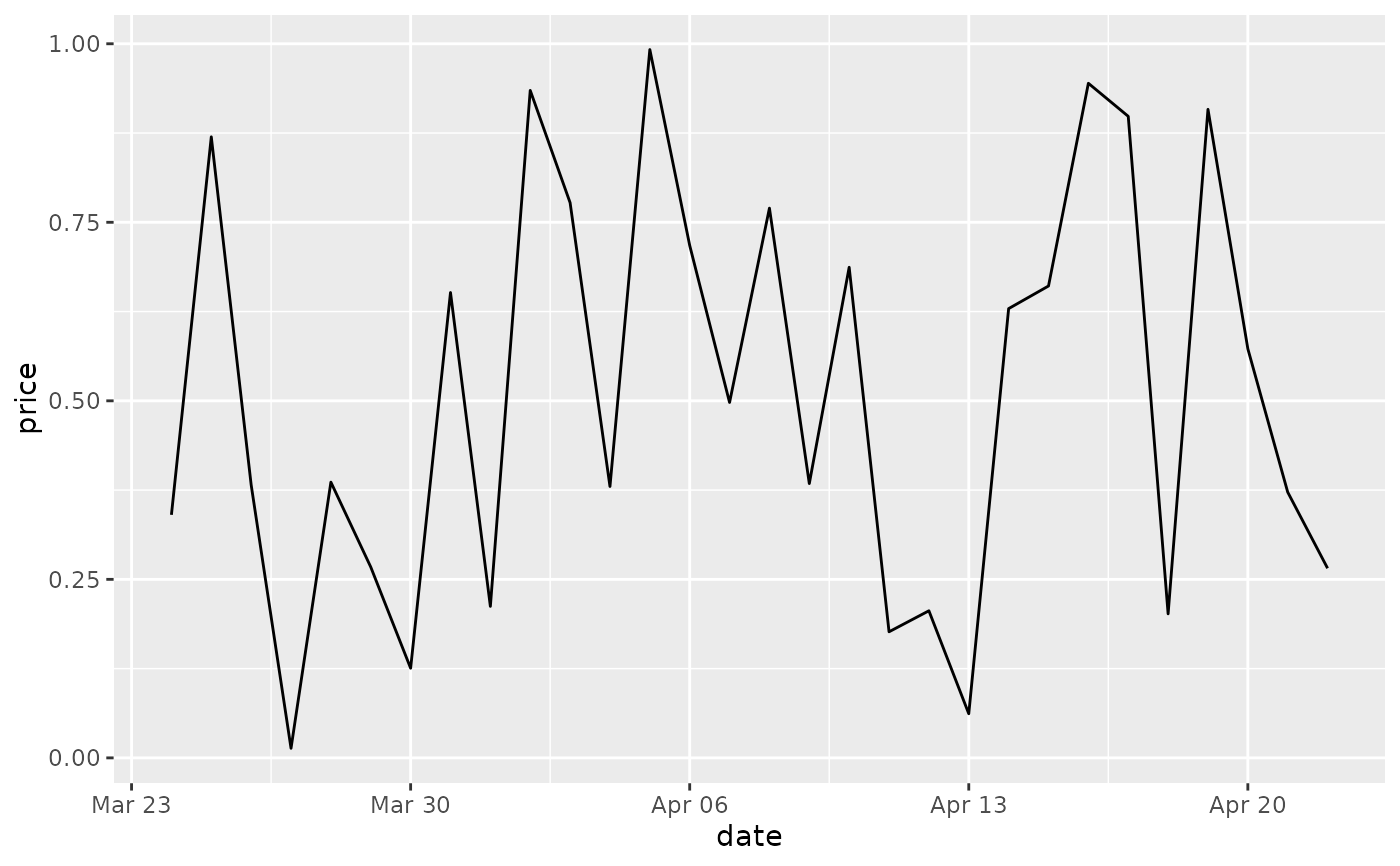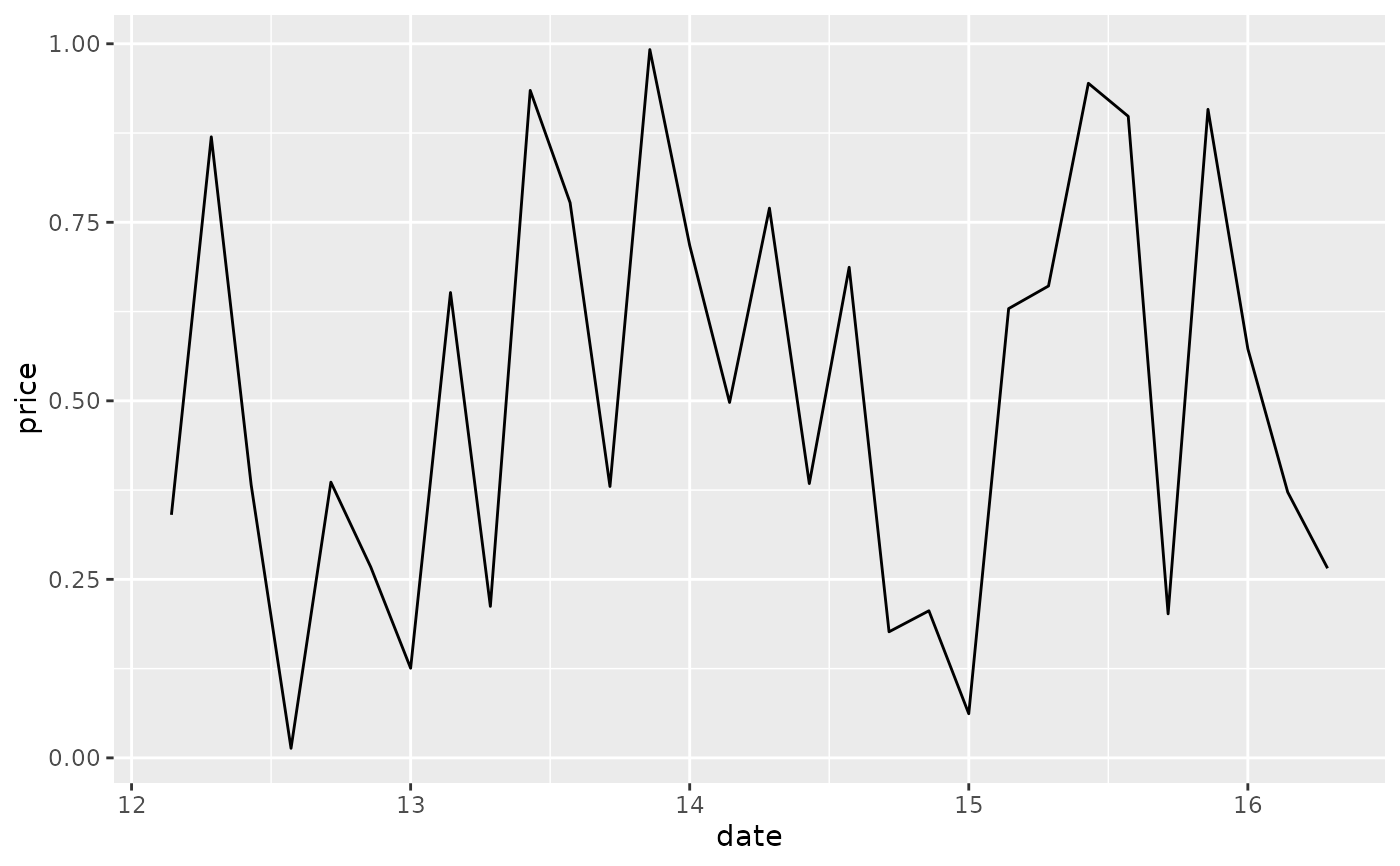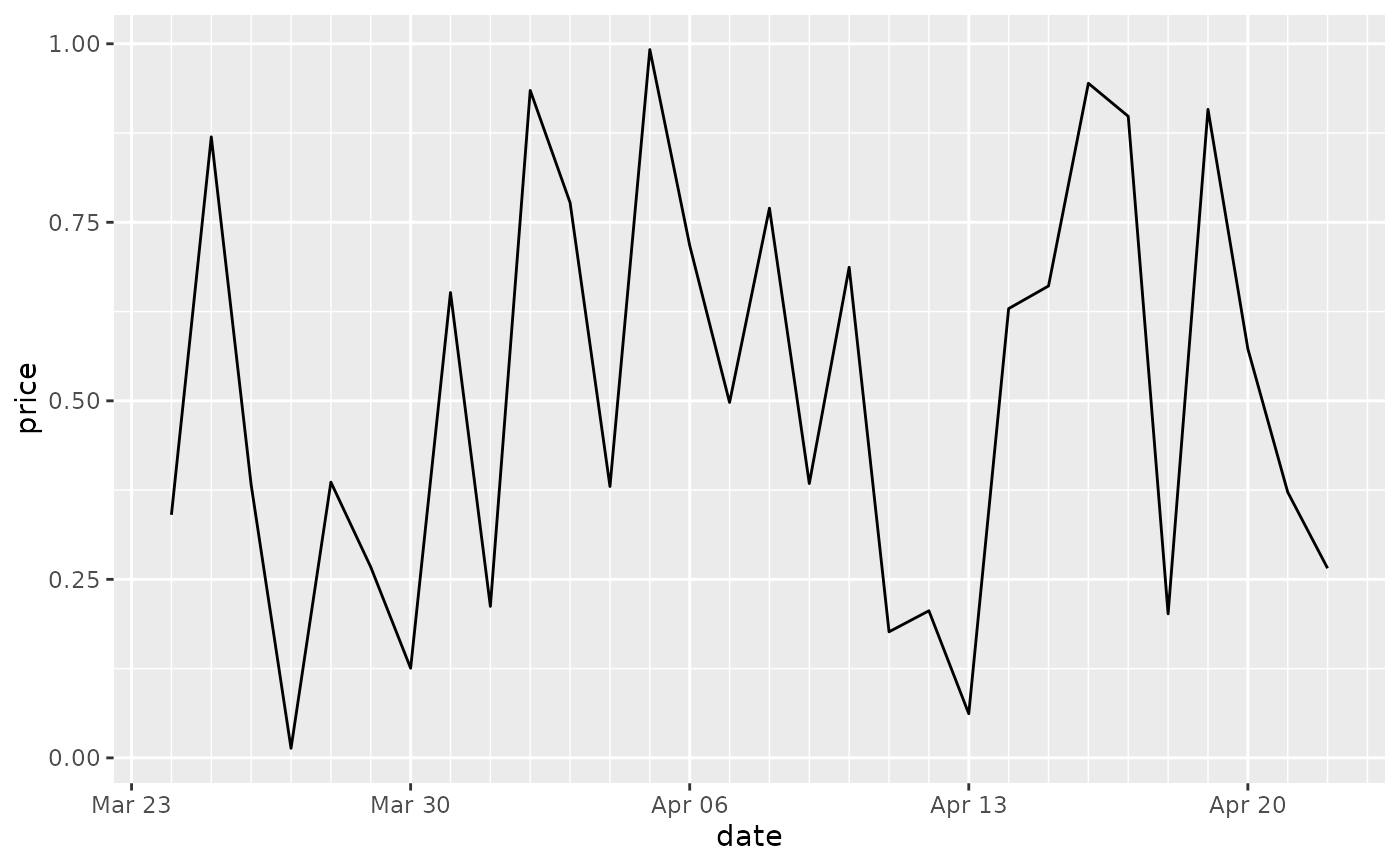These are the default scales for the three date/time class. These will usually be added automatically. To override manually, use scale_*_date for dates (class Date), scale_*_datetime for datetimes (class POSIXct), and scale_*_time for times (class hms).

scale_x_date(
name = waiver(),
breaks = waiver(),
date_breaks = waiver(),
labels = waiver(),
date_labels = waiver(),
minor_breaks = waiver(),
date_minor_breaks = waiver(),
limits = NULL,
expand = waiver(),
oob = censor,
guide = waiver(),
position = "bottom",
sec.axis = waiver()
)

scale_y_date(
name = waiver(),
breaks = waiver(),
date_breaks = waiver(),
labels = waiver(),
date_labels = waiver(),
minor_breaks = waiver(),
date_minor_breaks = waiver(),
limits = NULL,
expand = waiver(),
oob = censor,
guide = waiver(),
position = "left",
sec.axis = waiver()
)

scale_x_datetime(
name = waiver(),
breaks = waiver(),
date_breaks = waiver(),
labels = waiver(),
date_labels = waiver(),
minor_breaks = waiver(),
date_minor_breaks = waiver(),
timezone = NULL,
limits = NULL,
expand = waiver(),
oob = censor,
guide = waiver(),
position = "bottom",
sec.axis = waiver()
)

scale_y_datetime(
name = waiver(),
breaks = waiver(),
date_breaks = waiver(),
labels = waiver(),
date_labels = waiver(),
minor_breaks = waiver(),
date_minor_breaks = waiver(),
timezone = NULL,
limits = NULL,
expand = waiver(),
oob = censor,
guide = waiver(),
position = "left",
sec.axis = waiver()
)

scale_x_time(
name = waiver(),
breaks = waiver(),
minor_breaks = waiver(),
labels = waiver(),
limits = NULL,
expand = waiver(),
oob = censor,
na.value = NA_real_,
guide = waiver(),
position = "bottom",
sec.axis = waiver()
)

scale_y_time(
name = waiver(),
breaks = waiver(),
minor_breaks = waiver(),
labels = waiver(),
limits = NULL,
expand = waiver(),
oob = censor,
na.value = NA_real_,
guide = waiver(),
position = "left",
sec.axis = waiver()
)

## Arguments

name The name of the scale. Used as the axis or legend title. If waiver(), the default, the name of the scale is taken from the first mapping used for that aesthetic. If NULL, the legend title will be omitted. One of: NULL for no breaks waiver() for the breaks specified by date_breaks A Date/POSIXct vector giving positions of breaks A function that takes the limits as input and returns breaks as output A string giving the distance between breaks like "2 weeks", or "10 years". If both breaks and date_breaks are specified, date_breaks wins. One of: NULL for no labels waiver() for the default labels computed by the transformation object A character vector giving labels (must be same length as breaks) A function that takes the breaks as input and returns labels as output. Also accepts rlang lambda function notation. A string giving the formatting specification for the labels. Codes are defined in strftime(). If both labels and date_labels are specified, date_labels wins. One of: NULL for no breaks waiver() for the breaks specified by date_minor_breaks A Date/POSIXct vector giving positions of minor breaks A function that takes the limits as input and returns minor breaks as output A string giving the distance between minor breaks like "2 weeks", or "10 years". If both minor_breaks and date_minor_breaks are specified, date_minor_breaks wins. One of: NULL to use the default scale range A numeric vector of length two providing limits of the scale. Use NA to refer to the existing minimum or maximum A function that accepts the existing (automatic) limits and returns new limits. Also accepts rlang lambda function notation. Note that setting limits on positional scales will remove data outside of the limits. If the purpose is to zoom, use the limit argument in the coordinate system (see coord_cartesian()). For position scales, a vector of range expansion constants used to add some padding around the data to ensure that they are placed some distance away from the axes. Use the convenience function expansion() to generate the values for the expand argument. The defaults are to expand the scale by 5% on each side for continuous variables, and by 0.6 units on each side for discrete variables. One of: Function that handles limits outside of the scale limits (out of bounds). Also accepts rlang lambda function notation. The default (scales::censor()) replaces out of bounds values with NA. scales::squish() for squishing out of bounds values into range. scales::squish_infinite() for squishing infinite values into range. A function used to create a guide or its name. See guides() for more information. For position scales, The position of the axis. left or right for y axes, top or bottom for x axes. sec_axis() is used to specify a secondary axis. The timezone to use for display on the axes. The default (NULL) uses the timezone encoded in the data. Missing values will be replaced with this value.

sec_axis() for how to specify secondary axes

Other position scales: scale_x_binned(), scale_x_continuous(), scale_x_discrete()

## Examples

last_month <- Sys.Date() - 0:29
df <- data.frame(
date = last_month,
price = runif(30)
)
base <- ggplot(df, aes(date, price)) +
geom_line()

# The date scale will attempt to pick sensible defaults for
# major and minor tick marks. Override with date_breaks, date_labels
# date_minor_breaks arguments.
base + scale_x_date(date_labels = "%b %d")base + scale_x_date(date_breaks = "1 week", date_labels = "%W")base + scale_x_date(date_minor_breaks = "1 day")# Set limits
base + scale_x_date(limits = c(Sys.Date() - 7, NA))
#> Warning: Removed 22 row(s) containing missing values (geom_path).